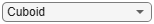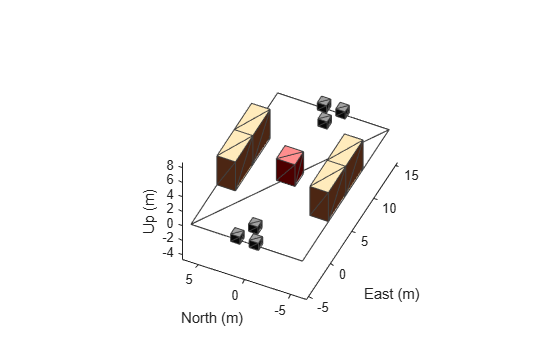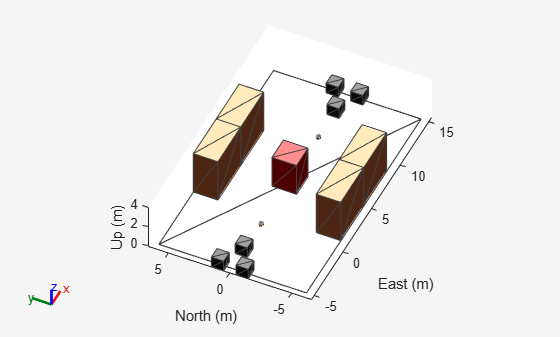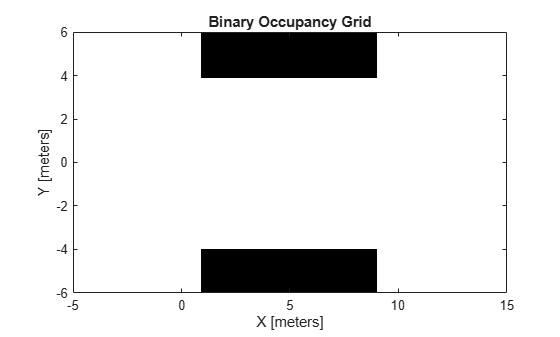# Perform Obstacle Avoidance in Warehouse Scenario with Mobile Robots

Create a scenario to simulate two mobile robots performing obstacle avoidance in a warehouse. This example demonstrates how to create a warehouse scenario, add mobile robots using the rigid body tree representation, model the kinematics of the robots, and simulate the behavior of the control algorithms using the resultant scenario.

### Create Warehouse Scenario

A `robotScenario` object consists of static meshes and `robotPlatform` objects. The `robotPlatform` objects can be static or movable. The `robotPlatform` object supports robot model specified as `rigidBodyTree` object, which enables SDF and URDF model support. In this example, the warehouse scenario can be created with static box meshes or with SDF models.

`scenario = robotScenario(UpdateRate=10);`

Add a plane mesh as ground plane in the scenario.

`addMesh(scenario,"Plane",Position=[5 0 0],Size=[20 12],Color=[0.7 0.7 0.7]);`

#### Create Warehouse Scenario Using Static Meshes

By default, `scenarioOptions` is set to `Cuboid`, here the warehouse scenario is constructed using static cuboid meshes. The cuboid meshes provides low fidelity simulation environment, which helps in testing algorithms with basic scenario elements.

```% Select warehouse scenario options. scenarioOptions ="Cuboid";```

In the warehouse scenario, the left and right side box meshes are considered as stationary shelves. So these areas are treated as restricted region for the robots and considered in the occupancy map, with `IsBinaryOccupied` parameter set to `true`.

```if strcmp(scenarioOptions,"Cuboid") addMesh(scenario,"Box",Position=[3 5 2],Size=[4 2 4],Color=[1 0.5 0.25],IsBinaryOccupied=true); addMesh(scenario,"Box",Position=[3 -5 2],Size=[4 2 4],Color=[1 0.5 0.25],IsBinaryOccupied=true); addMesh(scenario,"Box",Position=[7 5 2],Size=[4 2 4],Color=[1 0.5 0.25],IsBinaryOccupied=true); addMesh(scenario,"Box",Position=[7 -5 2],Size=[4 2 4],Color=[1 0.5 0.25],IsBinaryOccupied=true);```

The robot loads and unloads objects from the loading and unloading positions. These objects are non-stationary and represented with static cuboid meshes. So these meshes are not considered in the occupancy map.

``` addMesh(scenario,"Box",Position=[-3 0 0.5],Size=[1 1 1],Color=[0.1 0.1 0.1]); addMesh(scenario,"Box",Position=[-5 1 0.5],Size=[1 1 1],Color=[0.1 0.1 0.1]); addMesh(scenario,"Box",Position=[-5 -1 0.5],Size=[1 1 1],Color=[0.1 0.1 0.1]); addMesh(scenario,"Box",Position=[13 0 0.5],Size=[1 1 1],Color=[0.1 0.1 0.1]); addMesh(scenario,"Box",Position=[15 1 0.5],Size=[1 1 1],Color=[0.1 0.1 0.1]); addMesh(scenario,"Box",Position=[15 -1 0.5],Size=[1 1 1],Color=[0.1 0.1 0.1]);```

The warehouse scenario also contains an unattended non-stationary object which is represented with static cuboid mesh. The robot treats this object as obstacle and avoids if found between planned paths. This scenario element is not considered in the occupancy map.

``` addMesh(scenario,"Box",Position=[5 0 1.5],Size=[2 2 3],Color=[1 0 0]); end```

#### Create Warehouse Scenario Using SDF Models

To get more realistic warehouse scenario, download robomaker warehouse models and set `scenarioOptions` to `SDF`. These SDF models are added as static `robotPlatform` objects to create the warehouse scenario. The SDF models provides high fidelity simulation environment.

Load the shelves, cluttering, and bucket SDF models as `rigidBodyTree` object.

```if strcmp(scenarioOptions,"SDF") shelfARBT = importrobot(fullfile(... "roboMaker","models","aws_robomaker_warehouse_ShelfD_01","model.sdf")); shelfBRBT = importrobot(fullfile(... "roboMaker","models","aws_robomaker_warehouse_ShelfE_01","model.sdf")); clutteringRBT = importrobot(fullfile(... "roboMaker","models","aws_robomaker_warehouse_ClutteringA_01","model.sdf")); bucketWareRBT = importrobot(fullfile(... "roboMaker","models","aws_robomaker_warehouse_Bucket_01","model.sdf"));```

The SDF models which represents shelves on left and right side of the warehouse, are considered as stationary. So these areas are treated as restricted region for the robots and considered in the occupancy map, with `IsBinaryOccupied` parameter set to `true`.

``` shelfAModel = robotPlatform("ShelfA",scenario,RigidBodyTree=shelfARBT,... InitialBasePosition=[3 4 0],IsBinaryOccupied=true); shelfCModel = robotPlatform("ShelfC",scenario,RigidBodyTree=shelfARBT,... InitialBasePosition=[3 -4 0],IsBinaryOccupied=true); shelfBModel = robotPlatform("ShelfB",scenario,RigidBodyTree=shelfBRBT,... InitialBasePosition=[7 4 0],IsBinaryOccupied=true); shelfDModel = robotPlatform("ShelfD",scenario,RigidBodyTree=shelfBRBT,... InitialBasePosition=[7 -4 0],IsBinaryOccupied=true);```

The cluttering SDF models are considered as loading and unloading boxes, which are accessed by robots. As these objects are non-stationary, these SDF models are not considered in the occupancy map.

``` loadingBoxModel = robotPlatform("LoadingBox",scenario,... RigidBodyTree=clutteringRBT,... InitialBasePosition=[-3 0 0],... InitialBaseOrientation=eul2quat([pi/2 0 0],"ZYX")); unloadingBoxModel = robotPlatform("UnloadingBox",scenario,... RigidBodyTree=clutteringRBT,... InitialBasePosition=[13 0 0],... InitialBaseOrientation=eul2quat([pi/2 0 0],"ZYX")); ```

The bucket SDF model is considered as unattended non-stationary object by the robots. The robot treats this object as obstacle and avoids if found between planned paths. This scenario element is not considered in the occupancy map.

``` unattendedBoxModel = robotPlatform("UnattendedBox",scenario, ... RigidBodyTree=bucketWareRBT,... InitialBasePosition=[5 0 0]); end```

Visualize the scenario.

```show3D(scenario); view(-65,45) light```### Add Mobile Robots to Scenario

This example models two mobile robots that are running obstacle avoidance algorithms. The robots will be moving between two specified positions in the warehouse.

```loadingPosition = [0 0]; unloadingPosition = [10 0];```

Load two AMR Pioneer 3DX mobile robot from the robot library as a `rigidBodyTree` object.

```[pioneerRBT,pioneerRBTInfo] = loadrobot("amrPioneer3DX"); ```

Add the two robot models specified as a `rigidBodyTree` object to the scenario using `robotPlatform` objects.

```robotA = robotPlatform("MobileRobotA",scenario,... RigidBodyTree=pioneerRBT,... InitialBasePosition=[loadingPosition,0]); robotB = robotPlatform("MobileRobotB",scenario,... RigidBodyTree=pioneerRBT,... InitialBasePosition=[unloadingPosition,0]);```

Visualize the scenario with the robots.

```show3D(scenario); view(-65,45) light```### Mount Lidar Sensor on Robots

A lidar sensor is mounted on each robot for obstacle detection.

Specify lidar sensor configurations.

```lidarConfig = struct(angleLower=-120,... angleUpper=120,... angleStep=0.2,... maxRange=3,... updateRate=2);```

Create lidar sensor model.

```lidarmodel = robotLidarPointCloudGenerator(... AzimuthResolution=lidarConfig.angleStep,... AzimuthLimits=[lidarConfig.angleLower,lidarConfig.angleUpper],... ElevationLimits=[0 1],... ElevationResolution=1,... MaxRange=lidarConfig.maxRange,... UpdateRate=lidarConfig.updateRate,... HasOrganizedOutput=true);```

Mount a sensor on each of the mobile robots.

```lidarA = robotSensor("LidarA",robotA,lidarmodel,MountingLocation=[0 0 0.2]); lidarB = robotSensor("LidarB",robotB,lidarmodel,MountingLocation=[0 0 0.2]);```

#### Sensor Data Visualization

During the simulation of the `robotScenario`, use the provided `plotFrames` output from the scene as the parent axes to visualize your sensor data in the correct coordinate frames.

```[ax,plotFrames] = show3D(scenario); view(-65,45) light```

Visualize the lidar sensor point cloud with scatter plot.

`[pointCloudA,pointCloudB] = exampleHelperInitializeSensorVisualization(ax,plotFrames);`### Plan Initial Paths for Robots

To get an initial plan, use the binary occupancy map extracted from the scenario. In `Cuboid` based scenario, left and right-side box meshes and in `SDF` based scenario, left and right-side shelf models are considered as static elements. Therefore, these areas are considered in the occupancy map, by enabling the `IsBinaryOccupied` parameter.

#### Get Binary Occupancy Map from Scenario

```map = binaryOccupancyMap(scenario,MapHeightLimits=[0 5],... GridOriginInLocal=[-5 -6],... MapSize=[20 12]);```

Visualize the 2-D binary occupancy map.

```figure; show(map)```#### Plan Initial Paths for Mobile Robots

Use the `plannerAStarGrid` planner to plan the paths for the robots with the knowledge of the obstacles known in prior. During the scenario simulation, the robots can react to other obstacles detected by the lidar sensors.

Store the grid locations of the loading and unloading positions on the map grid.

```loadingGridLocation = world2grid(map,loadingPosition); unloadingGridLocation = world2grid(map,unloadingPosition);```

```plannerA = plannerAStarGrid(map); plannedGridPathA = plan(plannerA,loadingGridLocation,unloadingGridLocation); plannedWorldPathA = grid2world(map,plannedGridPathA);```

```plannerB = plannerAStarGrid(map); plannedGridPathB = plan(plannerB,unloadingGridLocation,loadingGridLocation); plannedWorldPathB = grid2world(map,plannedGridPathB);```

### Create Kinematic Motion Model for Robots

The AMR Pioneer 3DX is a small differential-drive robot whose kinematic motion model is modeled using the `differentialDriveKinematics` object. Since this robot model is from the robot library, the kinematic models parameters are pre-defined. Modify the motion inputs and wheel speed range of the vehicle.

```% Specify kinematic motion model for robot A. robotDiffA = copy(pioneerRBTInfo.MobileBaseMotionModel); robotDiffA.VehicleInputs = "VehicleSpeedHeadingRate"; robotDiffA.WheelSpeedRange = [-Inf Inf]; % Specify kinematic motion model for robot B. robotDiffB = copy(robotDiffA);```

### Get Start and Goal Poses for Simulation

The current pose of each mobile robot is initialized with first waypoint from the planned path. The goal pose of each mobile robot is the last waypoint from the planned path.

```% Get Start and Goal Poses for robot A. robotAStartPosition = plannedWorldPathA(1,:); robotAGoalPosition = plannedWorldPathA(end,:); robotAInitialOrientation = 0; robotACurrentPose = [robotAStartPosition,robotAInitialOrientation]'; % Get Start and Goal Poses for robot B. robotBStartPosition = plannedWorldPathB(1,:); robotBGoalPosition = plannedWorldPathB(end,:); robotBInitialOrientation = 0; robotBCurrentPose = [robotBStartPosition,robotBInitialOrientation]';```

### Create Controllers for Path Following and Obstacle Avoidance

Use `controllerPurePursuit` motion controller to make the robot follow the planned path.

```% Setup controllerPurePursuit for robot A. controllerA = controllerPurePursuit; controllerA.Waypoints = plannedWorldPathA; controllerA.DesiredLinearVelocity = 0.6; controllerA.MaxAngularVelocity = 2; controllerA.LookaheadDistance = 0.3; % Setup controllerPurePursuit for robot B. controllerB = controllerPurePursuit; controllerB.Waypoints = plannedWorldPathB; controllerB.DesiredLinearVelocity = 0.6; controllerB.MaxAngularVelocity = 2; controllerB.LookaheadDistance = 0.3;```

To react to obstacles in the path, the robots need to be equipped with an obstacle avoidance algorithm. The `controllerVFH` computes steering angle based on the inputs from a lidar sensor.

```% Setup controllerVFH for robot A. vfhA = controllerVFH; vfhA.UseLidarScan = true; vfhA.SafetyDistance = 1; % Setup controllerVFH for robot B. vfhB = controllerVFH; vfhB.UseLidarScan = true; vfhB.SafetyDistance = 1;```

### Simulate Robots in Scenario

Now that the warehouse scenario has been configured, visualize the original planned paths and then simulate the actual behavior of the mobile robots in the scenario.

Start by overlaying the original planned paths on the scenario. Observe how the robot moves with regards to the unexpected obstacle.

```hold(ax,"on") % Visualize planned path for robot A. plannedPathA = plot(ax,plannedWorldPathA(:,1),plannedWorldPathA(:,2),"-bs",... LineWidth=1,... MarkerSize=1.5,... MarkerEdgeColor="b",... MarkerFaceColor=[0.5 0.5 0.5]); % Visualize planned path for robot B. plannedPathB = plot(ax,plannedWorldPathB(:,1),plannedWorldPathB(:,2),"-cs",... LineWidth=1,... MarkerSize=1.5,... MarkerEdgeColor="b",... MarkerFaceColor=[0.5 0.5 0.5]); hold(ax,"off")```

Set up and run the simulation.

```setup(scenario) robotAReached = false; robotBReached = false; stopSimulation = false; while ~stopSimulation if ~robotAReached % Get current pose of the robot A, along with lidar sensor point % cloud till robot reaches to destination. [robotACurrentPose, isUpdatedA, robotAReached, pointCloudA] = ... exampleHelperGetCurrentPose(controllerA, robotDiffA, vfhA, lidarA, ... lidarConfig, robotACurrentPose, robotAGoalPosition, robotAReached); end if ~robotBReached % Get current pose of the robot B, along with lidar sensor point % cloud till robot reaches to destination. [robotBCurrentPose, isUpdatedB, robotBReached, pointCloudB] = ... exampleHelperGetCurrentPose(controllerB, robotDiffB, vfhB, lidarB, ... lidarConfig, robotBCurrentPose, robotBGoalPosition, robotBReached); end % Stop simulation if both robots reached to the destination. if robotAReached && robotBReached stopSimulation = true; end % Update plot when lidar sensor readings are updated. if isUpdatedA || isUpdatedB % Use fast update to move platform visualization frames. show3D(scenario,FastUpdate=true,Parent=ax); % Refresh all plot data and visualize. refreshdata drawnow limitrate end if ~robotAReached % Move robot A with current robot pose. move(robotA,"base",[robotACurrentPose(1), robotACurrentPose(2), 0, ... zeros(1,6), eul2quat([robotACurrentPose(3), 0,0]),... zeros(1,3)]); hold(ax,"on") % Visualize the path followed by Robot A. followedPathA = plot(ax,robotACurrentPose(1),robotACurrentPose(2),"-gs",... LineWidth=1,... MarkerSize=1.5,... MarkerEdgeColor="g",... MarkerFaceColor=[0.5 0.5 0.5]); hold(ax,"off") end if ~robotBReached % Move robot B with current robot pose. move(robotB,"base",[robotBCurrentPose(1), robotBCurrentPose(2), 0, ... zeros(1,6), eul2quat([robotBCurrentPose(3), 0,0]),... zeros(1,3)]); hold(ax,"on") % Visualize the path followed by Robot B. followedPathB = plot(ax,robotBCurrentPose(1),robotBCurrentPose(2),"-rs",... LineWidth=1,... MarkerSize=1.5,... MarkerEdgeColor="r",... MarkerFaceColor=[0.5 0.5 0.5]); hold(ax,"off") end hold(ax,"on") legend(ax,[plannedPathA, plannedPathB, followedPathA, followedPathB],... ["Planned Path for RobotA","Planned Path for RobotB",... "Path Followed by RobotA","Path Followed by RobotB"]) hold(ax,"off") % Advance scenario simulation time. advance(scenario); % Update all sensors in the scenario. updateSensors(scenario); end```